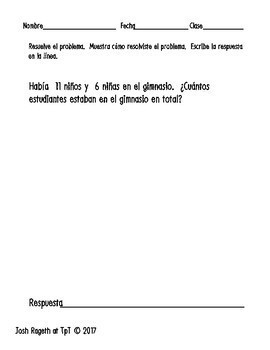# Spanish Differentiated Word Problems for Kindergarten, 1st & 2nd gradeSubject
Resource Type
File Type
Zip (566 KB|101 pages)
Standards
\$1.60
List Price:
\$2.00
You Save:
\$0.40
\$1.60
List Price:
\$2.00
You Save:
\$0.40
• Product Description
• Standards

This 100 page document contains 20 CGI questions IN SPANISH! As a bilingual / dual language / dual immersion educator, I have always had a hard time finding word problems in Spanish. Each question was designed with addition/subtraction levels in mind for 5 levels of differentiation. The students all get to work on the same problem with different numbers!

Each problem has a page with:

- numbers within 5

- numbers within 10

- numbers within 20

- 2 digit numbers with no regrouping / carrying

- blanks instead of numbers for teachers to differentiate for their own purposes

Problem types include:

Join/Separate

-start unknown

-result unknown

-change unknown

-part part whole

You May Also Like:

Bundle Adding / Subtracting with tens and ones and with the 100 Chart

Adding / Subtracting with a 100 chart / Sumas y restas con la tabla de 100

12 Mixed Word Problems / Problemas orales

How to Use This Resource:

• whole group
• small group
• special education (SPED)
• bilingual or Dual Language / DLI classrooms
• with ELLs for ESL instruction
• Great for K, 1st, 2nd, or 3rd grade

✰ ✭ ✰ ✭ ✰ ✭ ✰ ✭ ✰ ✭ ✰ ✭ ✰ ✭ ✰ ✭ ✰ ✭ ✰ ✭

You May Also Like:

Comprehensive Literacy Language Workshop Plan for Oliver Button is a Sissy

Dual Language Reading Resource using CAFE and Sentence Starters

Fluently add and subtract within 20 using mental strategies. By end of Grade 2, know from memory all sums of two one-digit numbers.
Use addition and subtraction within 100 to solve one- and two-step word problems involving situations of adding to, taking from, putting together, taking apart, and comparing, with unknowns in all positions, e.g., by using drawings and equations with a symbol for the unknown number to represent the problem.
Determine the unknown whole number in an addition or subtraction equation relating three whole numbers. For example, determine the unknown number that makes the equation true in each of the equations 8 + ? = 11, 5 = ▯ - 3, 6 + 6 = ▯.
Add and subtract within 20, demonstrating fluency for addition and subtraction within 10. Use strategies such as counting on; making ten (e.g., 8 + 6 = 8 + 2 + 4 = 10 + 4 = 14); decomposing a number leading to a ten (e.g., 13 - 4 = 13 - 3 - 1 = 10 - 1 = 9); using the relationship between addition and subtraction (e.g., knowing that 8 + 4 = 12, one knows 12 - 8 = 4); and creating equivalent but easier or known sums (e.g., adding 6 + 7 by creating the known equivalent 6 + 6 + 1 = 12 + 1 = 13).
Use addition and subtraction within 20 to solve word problems involving situations of adding to, taking from, putting together, taking apart, and comparing, with unknowns in all positions, e.g., by using objects, drawings, and equations with a symbol for the unknown number to represent the problem.
Total Pages
101 pages